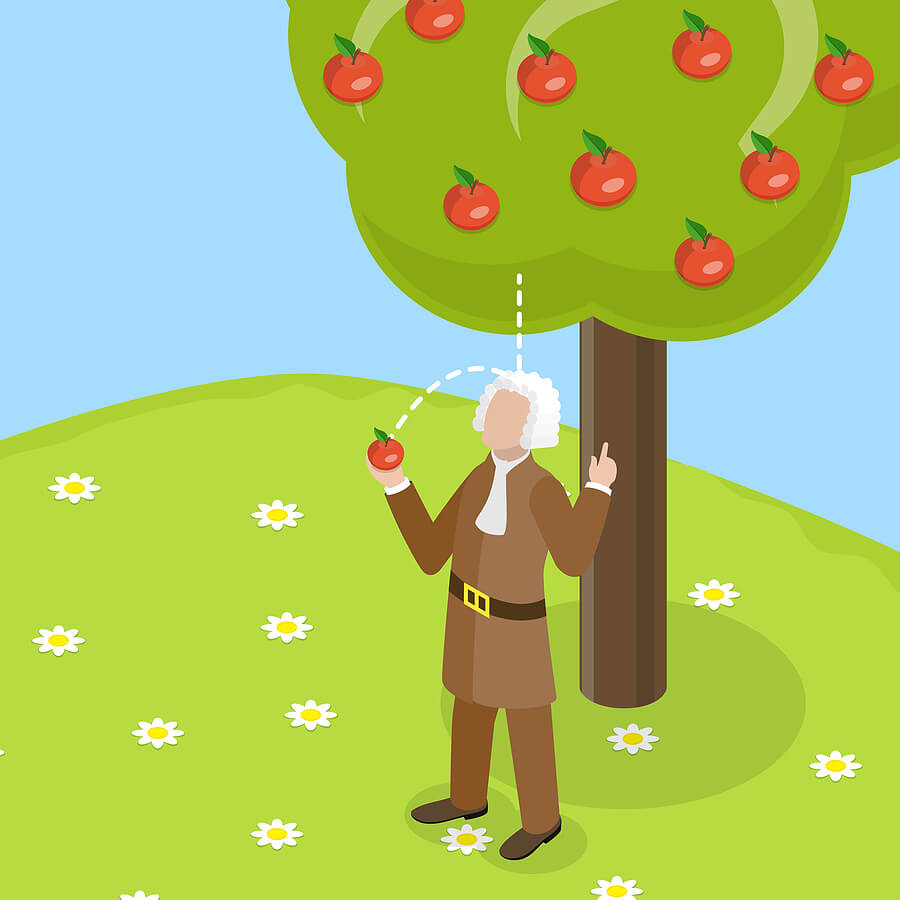# Understanding How Gravity Works Despite Differing WeightThe universal law of gravitation was discovered in the 1660s when an apple fell from a tree onto Isaac Newton’s head. While the story will never be proven to be true, one vital thing is the existence of a force that made the apple fall towards the ground, which Isaac Newton referred to as gravity.

What is the universal law of gravitation?

Newton’s universal law of gravitation states that any two items or bodies within the universe will attract one another with a force that is inversely proportional to the distance square between them and directly proportional to their masses.

According to the universal law of gravitation’s formula, we know that the only constant factor when measuring the force of attraction between the two bodies is known as the gravitational constant. Therefore, the force of attraction between the two bodies varies according to each mass and the distance of separation between the two bodies.

With that being said, how is it possible for everybody to fall at the same rate, no matter how high up they are or how heavy they are?

Earth’s gravity

The answer is actually quite obvious if we begin to consider the universal law of gravitation in Earth’s context. The Earth’s mass is approximately 5.972x1024 kg. Even if we talk about the heaviest projectile man has ever made, it pales in comparison at approximately 30,000kg. Hence, in the calculation of the universal law of gravitation, if we were to compare the two masses, we would realise the absurd difference. The only force that is exerting effectively is Earth’s gravity.

Weight and mass

Depending on each planet’s mass, gravity might differ. The moon’s gravity is approximately 1/6th of Earth’s. Hence, any object’s weight becomes 1/6th of what it will weigh on Earth as compared to the moon.

In the context of the Earth’s gravity, if we were to drop a rubber ball and a feather from the same height, it would be obvious that the rubber ball would hit the ground first. There are many factors that affect the rate they fall. For once, the feather’s low mass and unique structure make it susceptible to air pressure force, which works in the opposite direction to Earth’s gravity. The rubber ball is too heavy to be affected by that force.

However, one thing we know is that they are too light to impact the Earth’s gravity in any way. Hence, the constant factor is Earth’s gravity of 9.8m/s2. Hence, the speed at which any bodies fall towards Earth’s surface is dependent on their original mass, which remains constant.

Conclusion

Gravity is not the same throughout Earth. For example, places with increased underground mass might experience stronger gravitational force than places with less underground mass. In addition, the equator experience smaller gravitational force as compared to both poles.

Gravity is everywhere, even affecting the falling of the rain. And that is why the study of gravity is such a profound topic. If you are interested in understanding more about the universal law of gravitational and how it affects different physics topics, then look no further at Physics Tuition. We offer interesting and practical lessons that spur the interest among our students. Find out more at http://www.physics.com.sg/.